Mobile QR Code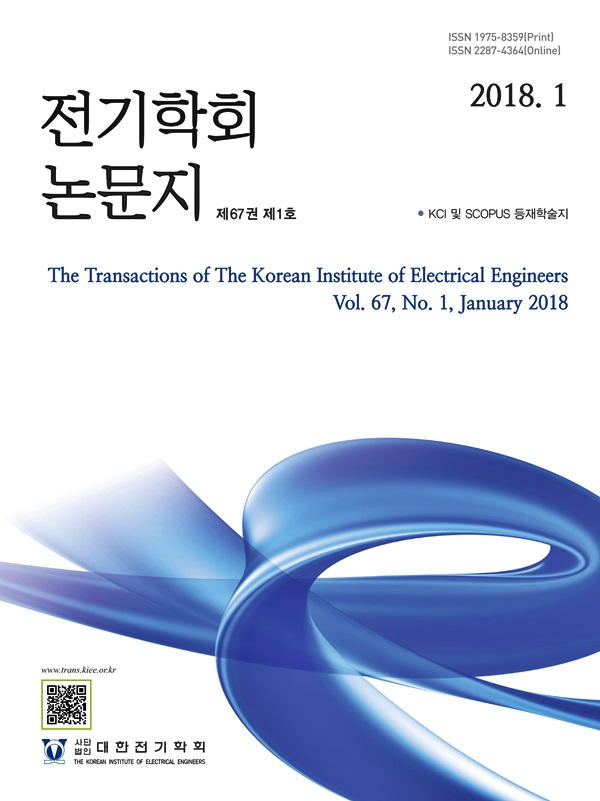#### The Transactions of the Korean Institute of Electrical Engineers

##### ISO Journal TitleTrans. Korean. Inst. Elect. Eng.
• SCOPUS
• KCI Accredited Journal

1. (Dept. of Electrical Engineering, Chosun University, Korea.)

Superconducting element, DC circuit breaker, LC circuit, Fault current, GdBCO

## 1. 서 론

As the IT and semiconductor industries increase, the demand for DC power is increasing. The current DC power grid is in the microgrid demonstration stageand it is the time when various factors are being studied at the same time. In the DC power system, MVDC and LVDC are being expanded from HVDCand research is being conducted to secure stability and reliability of the system. Therefore, we are conducting research on DC blocking technology to protect the peripheral devices of the DC system from line transients and to improve the reliability of DC system operation. We proposed a mechanical DC circuit breaker combining a superconducting element. This is due to the quenching of the superconducting element which can slow the growth rate of the initial fault currentand lead to a reliable cut-off operation through a mechanical DC circuit breaker. The mechanical DC circuit breaker consists of a mechanical DC switch, an LC divergence oscillation circuitand a SA. In this paper, the operating characteristics of a DC circuit breaker combined with a superconducting element were analyzed through experimentsand the characteristics generated in each element were compared and analyzed. And, we compared and analyzed the operating characteristics generated by giving the applied voltage as a variable of 300, 400, and 500 V. This was to check the initial fault current for each element according to the voltage/current level of the superconducting DC circuit breaker, the variable of the voltage/current according to the contects of the DC citcuit breaker opening operation, the time of the artificial zero-point generated and the completion time of the cut-off operation.

그림 1 초전도 DC 차단기의 등가 회로도

Fig. 1 Equivalent circuit diagram of a superconducting DC circuit breaker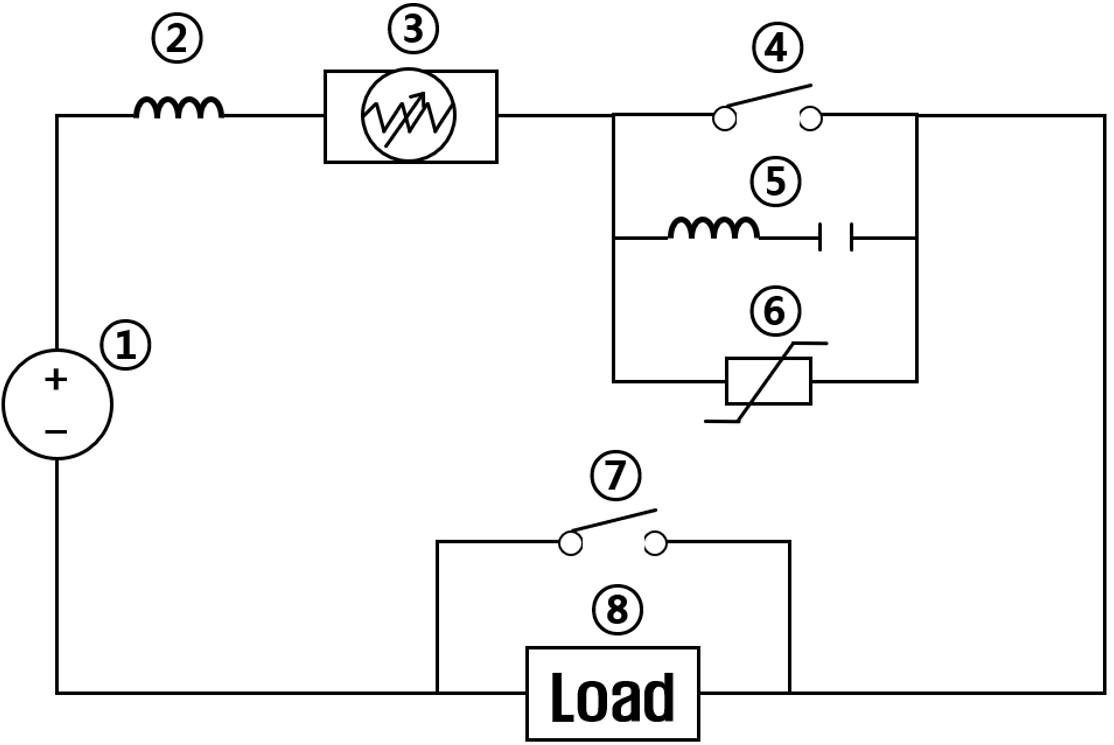그림 2 초전도 선재 (GdBCO)

Fig. 2 Superconducting wire (GdBCO)## 2. The superconducting DC circuit breaker

### 2.1 Configuration and mechanism

Figure 1 is an equivalent circuit diagram of a superconducting DC circuit breaker. Figure 1-① is DC power, ② is line impedanceand ③ is superconducting element. In a mechanical DC circuit breaker, the elements in Figure 1-④~⑥ are connected in parallel. Figure 1-④ is a mechanical DC switch, ⑤ is an LC divergence vibration circuitand ⑥ is a SA. Figure 1-⑦ is an accident switchand ⑧ is a load. In the normal state, the normal current flows from ① through ②, ③ and ④ to ⑧. In particular, the superconducting element ③ maintains a superconducting state that is cooled by 77K of liquid nitrogen. When the superconductor reaches the superconducting state, the resistance within the conductor changes to zero. Therefore, when the normal current passes through the superconducting element, it flows smoothly without being disturbed. And the mechanical DC switch ④ is closedand accident switch ⑦ is open. In the case of a transient state, accident switch ⑦ is closed so that a short circuit accident can be simulated. When a fault current occurs in the line, a fault current approximately 10 times higher than the normal current flowing in the line. The superconducting element reacts to the fault current within about 2 ms. When the state exceeds the critical point in the superconducting state, it changes into normal conduction within a short period of time and has its own resistance. Accordingly, the fault current is limited by the superconducting element within about 2 ms. The limited fault current is interrupted by the mechanical DC circuit breaker after the superconductor is quenched. First, the mechanical DC switch ④ is opened. Accordingly, the arc surge and the arc plasma are generated between the contacts. At the same time, in LC divergence oscillation circuit ⑤, divergent vibration is generated by arc. Accordingly, the fault current is affected. The fault current reaches an artificial zero point through an unspecified amplitude under the influence of the LC divergence oscillation circuit. When the fault current reaches the artificial zero point, the mechanical DC switch ④ completes the open operation. After that, the residual fault current remaining on the line is extinguished through the SA ⑥(1-4).

그림 3 시간에 따른 초전도 퀜칭 동작 특성 그래프 ⒜ 65 ms, ⒝ 4.5 ms

Fig. 3 The graph of operation characteristics of the superconducting quenching by applied time ⒜ 65 ms, ⒝ 4.5 ms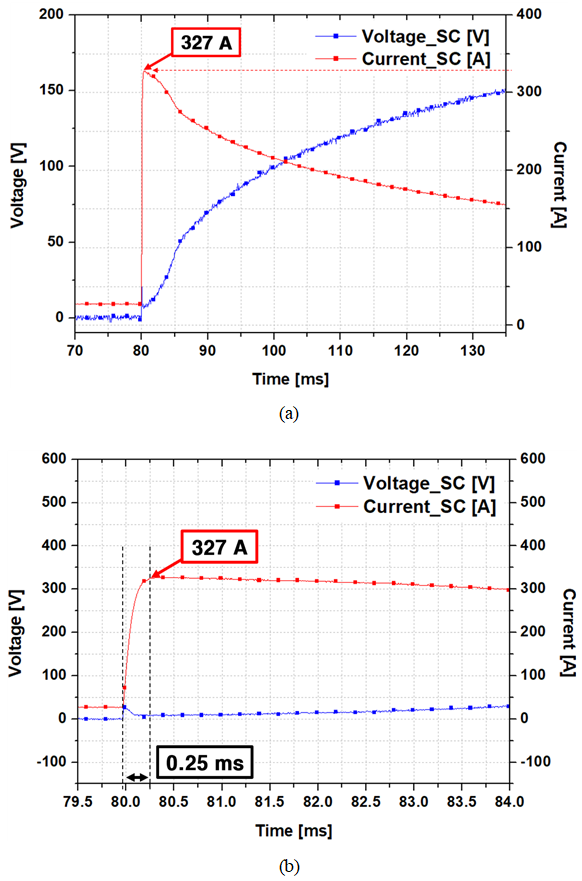### 2.2 The characteristics of the GdBCO supercon- ducting wire

Figure 2 is a GdBCO superconducting wire. High-temperature superconductors are manufactured in the form of Oxides of rare earth elements $REBa_{2}Cu_{3}O_{7-y}$. The most representative superconductor is $YBa_{2}Cu_{3}O_{7-y}$. YBCO superconductor is economical and the manufacturing process is simple. In addition, the critical current density is high in a magnetic fieldand it can be processed in the form of a thin film or a wire(5-8). The total length is about 5 m, the width of the wire is about 4 mmand the thickness is about 4.1 mm. The purpose of the experiment is to design a scale-down model at the voltage level of a real DC system and see the operating characteristics. Therefore, referring to this point, a superconducting wire with a critical current of about 200 [± 10%] A was selected. This showed about 1.8 times higher stability and faster quenching operation characteristics based on 1.0 μm thickness than the material of YBCO used in previous studies(5-7). Figure 3 is graphs of the quenching characteristics of the superconducting element GdBCO. Figure 3-⒜ is characteristic graph over time from 70 ms to 135 ms (total 65 ms)and Figure 3-⒝ is characteristic graph over time from 79.5 ms to 84.0 ms (total 4.5 ms). The applied voltage was about 300 Vand the time of the accident was about 80.0 ms.

그림 4 초전도 소자를 결합한 DC 차단기 동작 특성 그래프 (인가전압 300 V)

Fig. 4 The graph of operation characteristics of the DC circuit breaker combined with superconducting element (applied voltage 300 V)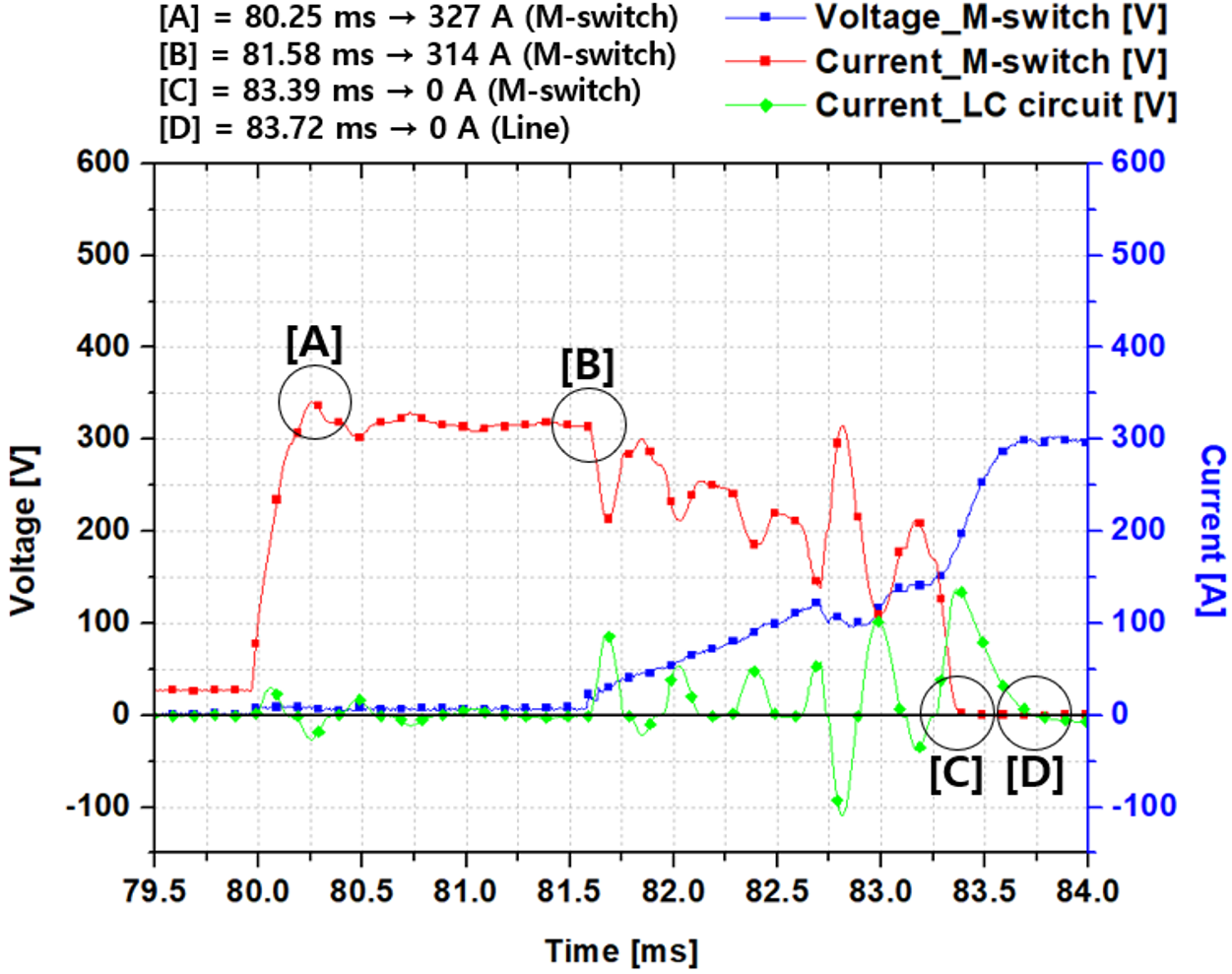Figure 3-⒜ shows the quenching of a superconducting device. The initial fault current was about 327A and it was about 152 A at about 135 ms. Figure 3-⒝ reduced the time according to the fast cut-off time condition of the DC breaker. It is graph showing the quenching operation characteristics of a superconducting element within about 4.5 ms.

그림 5 초전도 선재 모델

Fig. 5 The model of the superconducting wire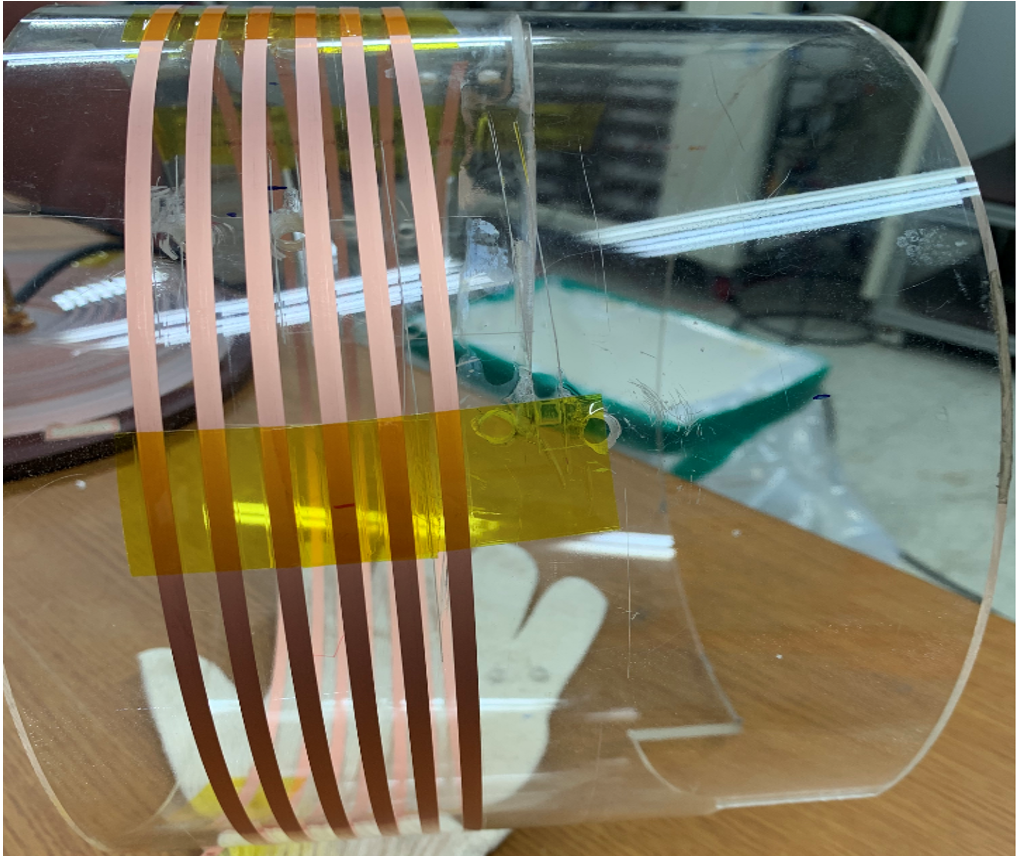그림 7 기계식 DC 스위치와 SA

Fig. 7 Mechanical DC switch and SA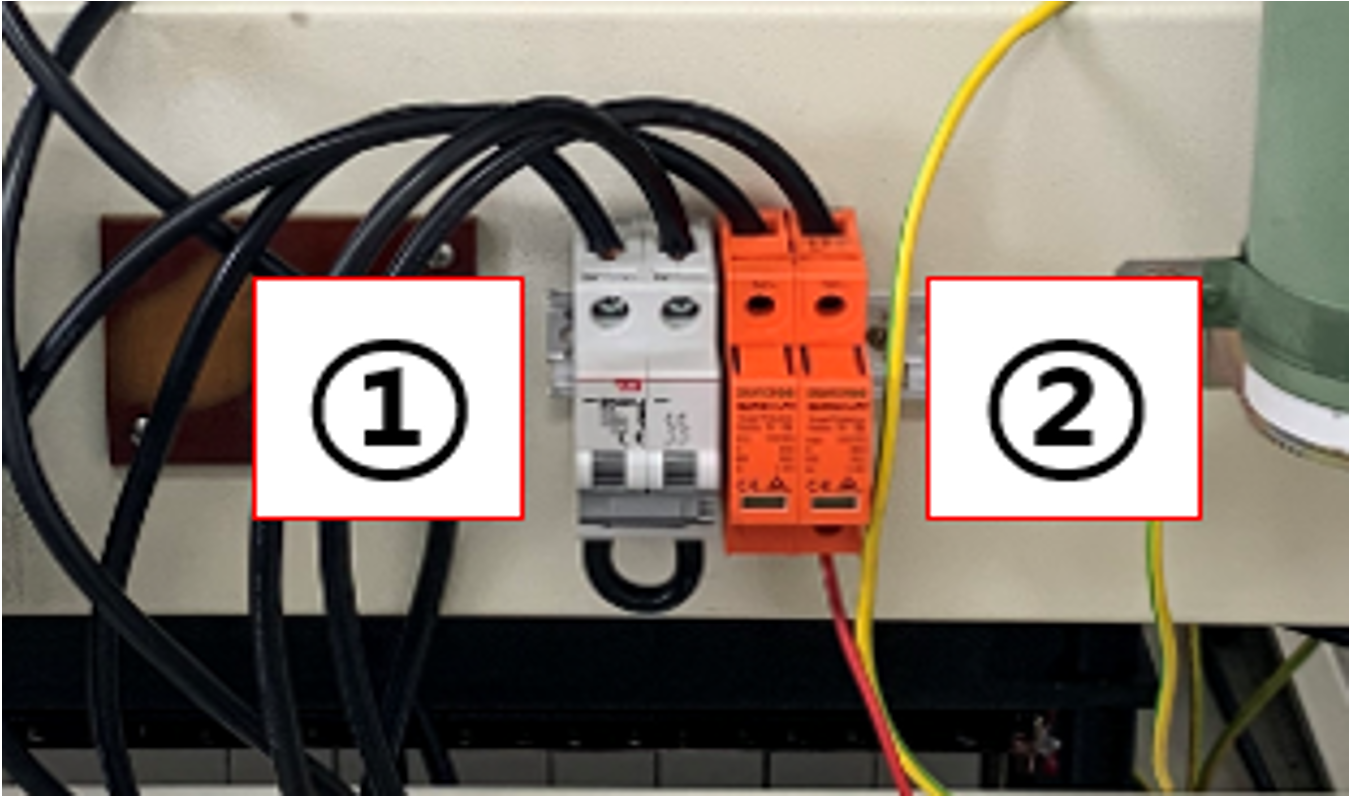그림 8 리액터와 커패시터 (LC 발산진동회로)

Fig. 8 Reactor and Capacitor (LC divergence oscillation circuit)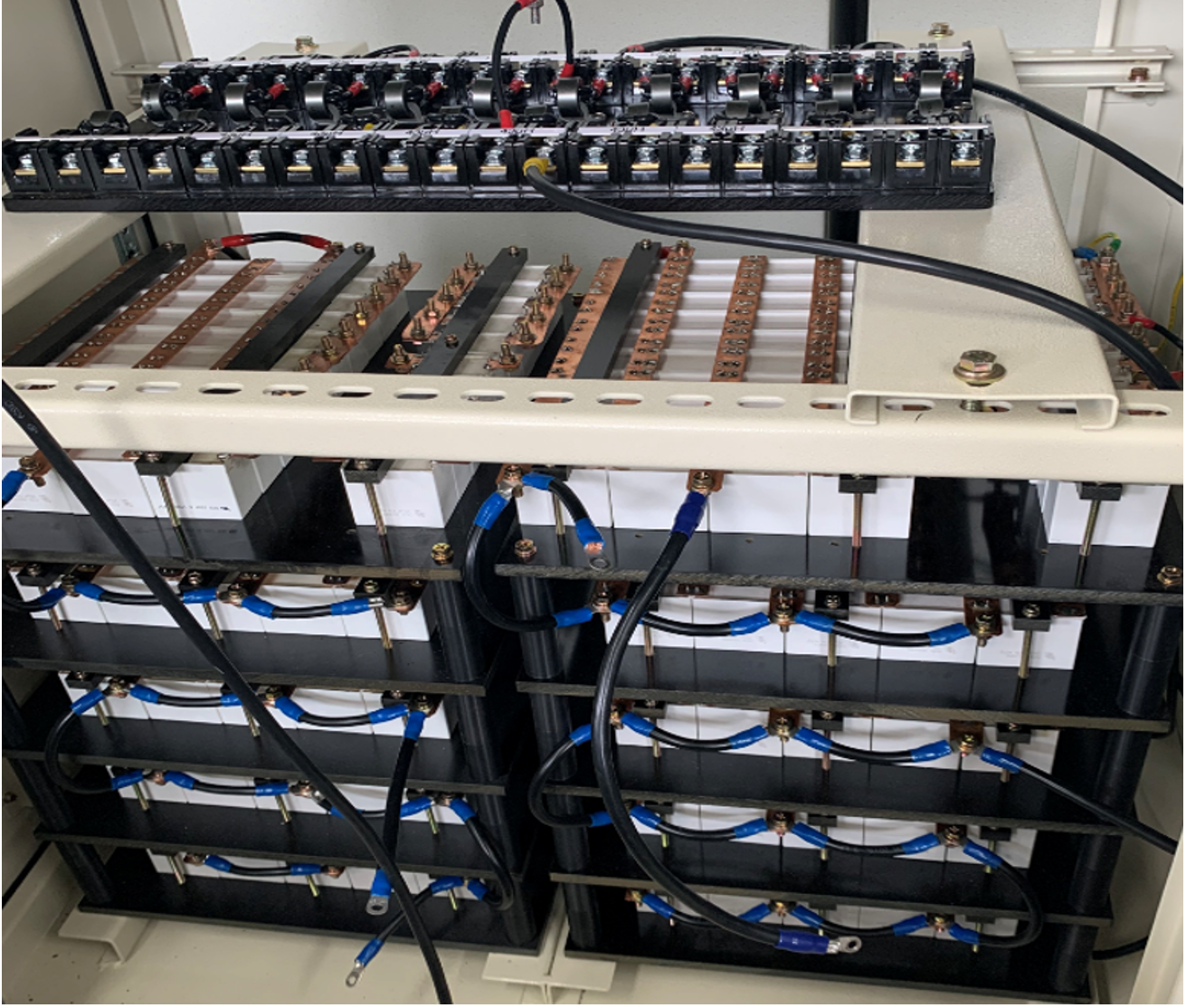Figure 4 is graph of the operating characteristics of a model that combined a superconducting element with a mechanical DC circuit breaker. A is the point at the initial fault current occuring. B is the point at the DC cut-off contacts are opening. C is the point at the artificial zero-point occuring. D is the point at the fault current is completely disappearing. At about 81.6 ms, the opening operation of the mechanical DC circuit breaker was made and the oscillation current was generated by the LC divergence oscillation circuit. The artificial zero point of the mechanical DC circuit breaker occurred at about 83.38 msand as a result, the fault current was extinguished at about 83.7 ms. Superconducting element had excellent ability to limit fault current, but could not cut-off fault current directly. The cut-off of fault current performed a mechanical DC circuit breaker. Among the components of a mechanical DC circuit breaker, the role of generating an artificial zero point of the fault current through the LC divergent oscillation circuit was also very important.

그림 9 기계식 DC 차단기의 임피던스의 동작 특성 그래프 (인가전압 500 V)

Fig. 9 The graph of the operation characteristics for the impedance in mechanical DC circuit breaker (applied voltage 500 V)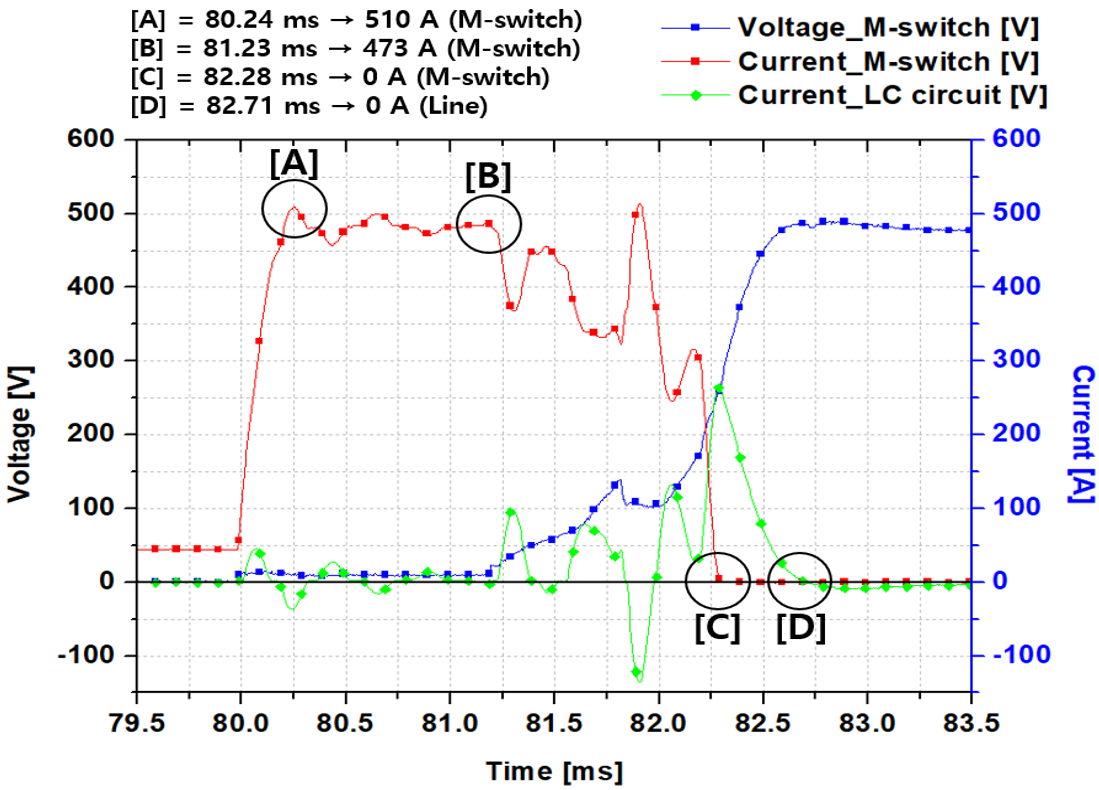### 2.3 Experimental Design

Figure 5 is a design model of a GdBCO superconducting wire wound around a circular acrylic contafiner. The pitch space for each wire is about 5 mm and a total wire of 5 m was used. In addition, the wire was fixed with Kapton tape to prevent vibration and shaking caused by the quenching operation of the superconducting wire. Figure 6 shows a DC power supply of about 12.4 V lead-acid batteries connected in series to control the desired voltage level. Figure 7 shows the disconnection switch ① and SA (surge arrester) ② of the mechanical DC circuit breaker. This mechanical DC switch is the BK63H model. We applied SA, which could act as a SA, to conduct an experiment at a low voltage level. Figure 8 is an LC divergence oscillation circuit in which a reactor and a capacitor are connected in series. In the experiment, the reactor was about 55 μm and the capacitor was about 205 μF. In this paper, after comparing and analyzing the operating characteristics according to the presence or absence of a superconducting element through an applied voltage of 500V, the applied voltage was increased to 600V and the same experiment was performed.

### 2.4 Experimental results

Figure 9 is graph of the operation characteristics of a mechanical DC circuit breaker without a superconducting element. The time point at which the fault occurred was about 80 msand the maximum value of the initial fault current occurred from about 80.24 ms to 510 A. The fault current was about 10 times higher for about 0.24 ms. The operation point of the DC circuit breaker started at about 81.23 msand this point is the same as the point at which the amplitude of the LC divergence oscillation circuit changes. The LC diverged oscillation current was about 98.47 A at about 81.3 ms, -136.95 A at about 81.9 msand about 265.43 A at about 82.28 ms. The resulting fault current was affectedand an artificial zero point was generated at about 82.28 ms. At this time, the opening operation of the mechanical DC switch was completed. After that, the residual current flowing in the LC divergence oscillation circuit is extinguished at about 82.71 ms by the SA. Therefore, the final cut-off time was about 2.71 ms.

그림 10 초전도 소자를 결합한 기계식 DC 차단기의 동작 특성 그래프 (인가전압 500 V)

Fig. 10 The graph of the operation characteristics combined superconducting element in mechanical DC circuit breaker (applied voltage 500 V)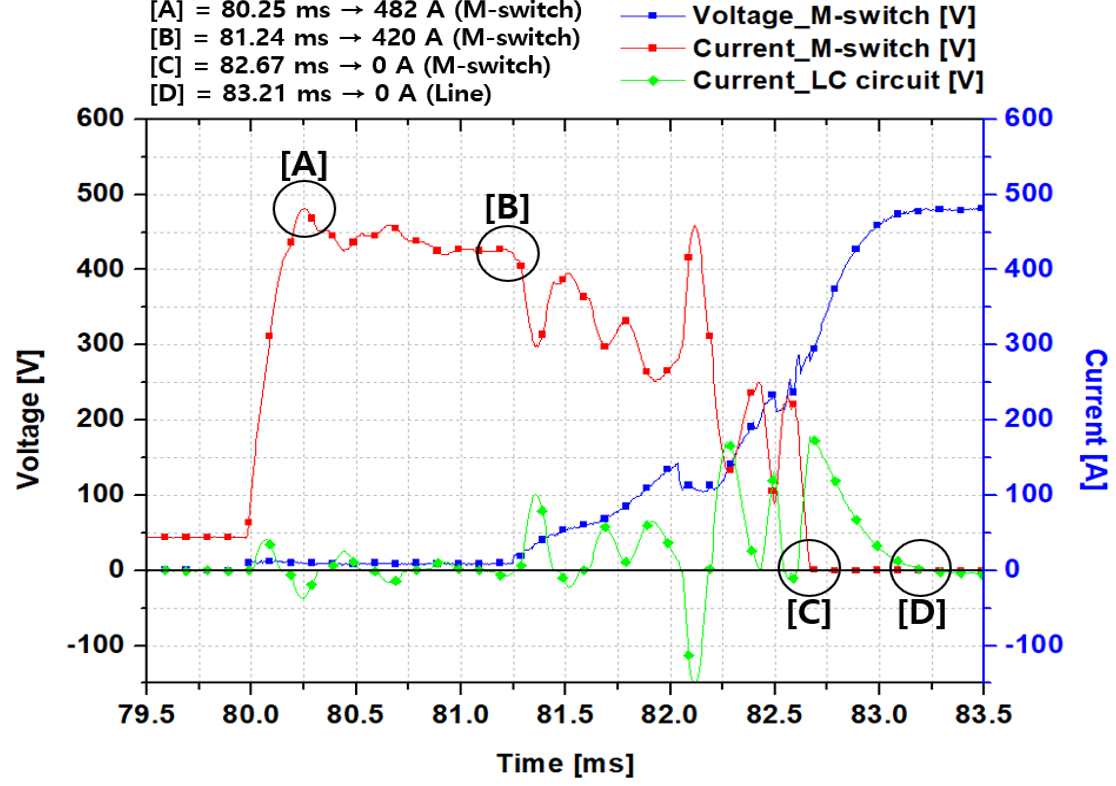Figure 10 is graph of the operation characteristics of a mechanical DC circuit breaker combined a superconducting element. The fault point is about 80.25 ms. The initial fault current was about 482 A at about 80.25 ms. It showed a current limiting rate of about 6 % by the superconducting element. The operation time of the DC circuit breaker was about 81.24 ms. After that, the LC divergence oscillation current was about 102.6 A at about 81.34 ms, about -150.8 A at 82.1 msand about 169.3 A at about 82.2 ms. Finally, the fault current reached an artificial zero at about 82.67 ms. It was confirmed that the artificial zero-point generation time of the fault current was delayed by about 0.39 ms depending on the presence or absence of the superconducting element. After this, the fault current was completely extinguished at about 83.21 ms by SA. The final cut-off time was about 3.21 ms.

그림 11 기계식 DC 차단기의 임피던스의 동작 특성 그래프 (인가전압 600 V)

Fig. 11 The graph of the operation characteristics for the impedance in mechanical DC circuit breaker (applied voltage 600 V)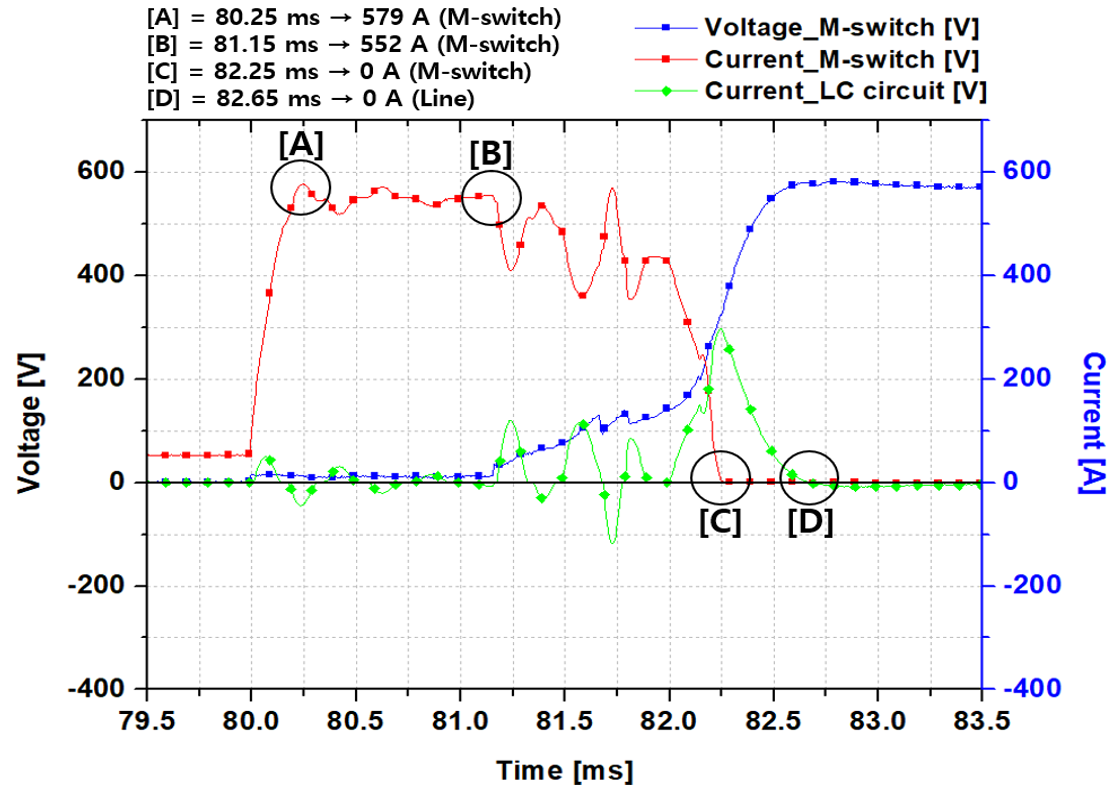Figure 11 is graph of the operating characteristics of a mechanical DC circuit breaker without a superconducting elementand the experiment was conducted at about 600 V by raising the applied voltage by 100 V. The time of the accident was the same as in the previous experiment. The initial fault current was about 579 A at about 80.25 ms. The opening operation of the mechanical DC switch occurred at about 81.15 msand it is the time when the LC divergence oscillation current occurs. The LC divergence oscillation current was about 121.6 A at about 81.23 ms, about -121.3 A at about 81.72 msand about 299.2 A at about 82.24 ms. The fault current flowing through the mechanical DC switch induced an artificial zero point at about 82.25 msand the opening operation was completed. The fault current remaining in the LC divergence oscillation circuit was extinguished at about 82.65 ms through the SA.

Figure 12 is graph of the operation characteristics of a superconducting mechanical DC circuit breaker that was experimented at 600 V of applied voltage. The time of the accident started at about 80 ms, it was the same as in the previous experiment. The initial fault current was about 540 A at about 80.25 ms. It showed a current limiting rate of about 6.8 % by the superconducting element. This is about 6.8 % higher current limiting rate than when the applied voltage was 500 V. The opening operation of the mechanical DC switch was started at about 81.18 ms. The LC divergence oscillation current was about 109.5 A at about 81.27 ms, about –164.3 A at 82.04 ms, 217.6 A at about 82.2 ms and -337.8 A at about 82.5 ms. The resulting fault current induced an artificial zero point at about 82.72 ms. At about 83.24 ms, the fault current was completely extinguished.

그림 12 초전도 소자를 결합한 기계식 DC 차단기의 동작 특성 그래프 (인가전압 600 V)

Fig. 12 The graph of the operation characteristics combined superconducting element in mechanical DC circuit breaker (applied voltage 600 V)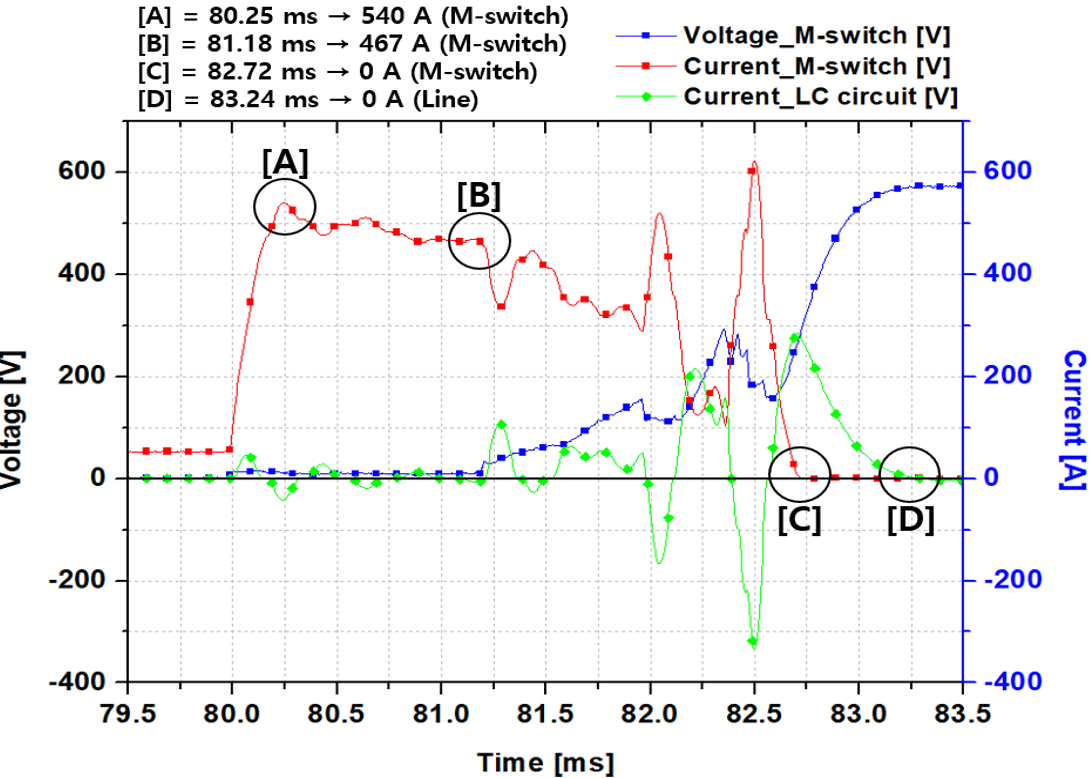## 3. Conclusion

In this paper, the operating characteristics of a mechanical DC circuit breaker according to the presence or absence of a superconducting element were compared and analyzed. In addition, by comparing and analyzing the operating characteristics according to the applied voltage, the characteristics of the initial accident current and the LC divergence oscillation current were analyzed. As a result, according to the application of the superconducting element, the increase of the initial fault current was suppressed and the power burden of the mechanical circuit breaker was reduced. When the voltage was raised, the current limiting rate of the superconducting element was confirmed to be higher. Additionally, the time at which the artificial zero point of the fault current is induced by the LC divergence oscillation circuit is also accelerated.

### References

1
H. Arai, M. Inaba, T. Ishigohka, H. Tanaka, K. Arai, M. Furuse, M. Umeda, 2006, Fundamental Characteristics of Superconducting Fault Current Limiter Using LC Resonance Circuit, IEEE Trans. Appl. Supercon., Vol. 16, No. 2, pp. 642-6452
S. Kawashima, T. Ishigohka, A. Ninomiya, M. Furuse, 2009, Power System Voltage Stabilizer Using LC Resonance, IEEE Trans. Appl. Supercon., Vol. 19, No. 3, pp. 2053-20563
S. H. Hwang, H. W. Choi, I. S. Jeong, H. S. Choi, 2018, Characteristics of DC Circuit Breaker Applying Transformer-Type Superconducting Fault Current Limiter, IEEE Trans. Appl. Supercon., Vol. 28, No. 4, pp. 1-54
H. W. Choi, I. S. Jeong, S. Y. Park, H. S. Choi, 2018, Characteristics of Superconducting Coil-Type DC Fault Current Limiter to Increase Stability in the Grid Connection PV Generation System, IEEE Trans. Appl. Supercon., Vol. 28, No. 3, pp. 1-45
M. Murakami, T. Oyama, H. Fujimoto, T. Taguchi, S. Gotoh, Y. Shiogara, N. Koshizuka, S. Tanaka, 1990, Large Levitation Force due to Flux Pinning in YBaCuO superconductors Fabricated by Melt-Powder-Melt Growth Process, Japanese J. Appl. Phys., Vol. 29, No. 11, pp. l1991-L19946
C. J. Kim, K. B. Kim, I. H. Kuk, G. W. Hong, Y. S. Lee, H. S. Park, 1997, Microstructure change during oxygen annealing and the effect on the levitation force of melt-textured Y-Ba-Cu-O superconductors, Supercond. Sci. Technol., Vol. 10, No. 12, pp. 947-9547
H. Techima, M. Sawamura, M. Morita, M. Tsuchimoto, 1997, Levitation forces of single-grained Y-Ba-Cu-O bulk superconductor of 48mm in diameter, Cryogenics, Vol. 37, No. 9, pp. 505-5098
D. A. Cardwell, N. Hari babu, 2006, Processing and properties of single grain (RE)-Ba-Cu-O bulk superconductors, Physica C, Vol. 445-448, pp. 1-7## 저자소개

Currently completing the PhD program at the same graduate school.

Regular member of the Korean Electrical Society

Major Research Areas of Interest : Applications of superconducting Power system, Applications of DC circuit breaker

Tel: 062-230-7054

E-mail : sangyong4400@gmail.com

Currently Professor, Department of Electrical Engineering, Chosun University, 2021~ Vice President of the Korean Electrical Society.

Major Research Areas of Interest : Applications of superconducting Power system, Applications of DC circuit breaker, wireless power transmission

Tel: 062-230-7025

E-mail : hyosang@chosun.ac.kr